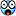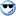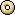A Flash Developer Resource Site

1. ## whats......

whats 2+2...?Reply With Quote

2. 4Reply With Quote

3. cool you guys really know yer stuffReply With Quote

4. Can anyone prove that 2+2=4?Reply With Quote

5. Originally posted by Sul
Can anyone prove that 2+2=4?
yes coz if you got 2 apples in front of you then you add another 2 apples then count them all you get 4 apples (its right coz ive just testedt it )Reply With Quote

6. Yeah that's great with apples
but what happens when you use peaches?

then the math gets fuzzy cause peaches have fuzz on them.Reply With Quote

7. true but if you wash the peaches then it all becomes as clear as the water used to wash themReply With Quote

8. x^2 = x*x = x+x+x+x+...(x times)

d/dx (x^2) = d/dx (x+x+x+...)
=> 2x = 1+1+1+...(x times)
=> 2x = x
=> 2x / x = x / x
=> 2 = 1

jReply With Quote

9.Reply With Quote

10. x^2 = x*x = x+x+x+x+...(x times)

d/dx (x^2) = d/dx (x+x+x+...)
=> 2x = 1+1+1+...(x times)
=> 2x = x
=> 2x / x = x / x
=> 2 = 1
HAHA! CAN'T DEVIDE BY X!!! WHAT IF X IS 0?!
You can prove that sheep can fly if you devide by zero!
And btw-

from d/dx (x^2) = d/dx (x+x+x+...) to 2x = 1+1+1+...(x times), something doesn't make any sense...

d/dx (x^2) = d/dx (x+x+x+...) /(d/dx)
x^2 = (x+x+x+...) /x <-- which CAN'T be done!
x = 1+1+1+...(x times) <-- now that makes sense!
x = x

but how did you get to:
2x= 1+1+1+...(x times)??Reply With Quote

11. Originally posted by halflifedarknes
HAHA! CAN'T DEVIDE BY X!!! WHAT IF X IS 0?!
You can prove that sheep can fly if you devide by zero!
And btw-

from d/dx (x^2) = d/dx (x+x+x+...) to 2x = 1+1+1+...(x times), something doesn't make any sense...

d/dx (x^2) = d/dx (x+x+x+...) /(d/dx)
x^2 = (x+x+x+...) /x <-- which CAN'T be done!
x = 1+1+1+...(x times) <-- now that makes sense!
x = x

but how did you get to:
2x= 1+1+1+...(x times)??
It uses derivatives (calculas)

x^2 = x*x = x+x+x+x+...(x times)
// x squared is x*x,
// 2*x is x+x(2 times)
// 3*x is x+x+x (3 times)
// so x*x is x+x+x...(x times)

d/dx (x^2) = d/dx (x+x+x+...)
//take the derivative of both sides
// derivative of x squared is 2*x
// derivative (x+x+x+...) is
// derivative of x + derivative of x + ...
// the derivative of x is 1, so derivative of x+x+x+...(x times) is
// 1+1+1+...(x times) = x

=> 2x = 1+1+1+...(x times)
=> 2x = x // true, only if x is zero, but x is a variable, it could have been 2, 3, 4...
=> 2x / x = x / x // so if x != 0
=> 2 = 1 // then 2 = 1

The trick isn't in the division, that actual problem lies in the fact that you can only take the derivative of a continuous expression (in this case), but here, it's implied, but not directly stated, that x must be a non-negative integer. If x = 0.5, then x^2 = x*x = x+x+x+...(x times) = 0.5 + 0.5 + ... (0.5 times) doesn;t make much sense. So x is not continuous and therefore the derivative cannot be taken giving us the falacy in the argument.

jReply With Quote

12. It uses derivatives (calculas)
So that's what it is...
I didn't know that it is written like that...
Sothat DOES makes sense, sorta... If X is an absolute number, and not 0...Reply With Quote

13. all this fuss for 2+2 lolReply With Quote

14. That is a cool trick yasunobu13. I'll remember that one.Reply With Quote

15. If a bear ****s in the woods, does it make a sound?Reply With Quote

16. ahh but do bears realy s**t in the woods..?Reply With Quote

17. SWARTSENAIGER FOR PRESIDENT !!!!!!!!!!!

FREE's to all the bears in the woods !Reply With Quote

####Posting Permissions

• You may not post new threads
• You may not post replies
• You may not post attachments
• You may not edit your posts
•

 » Home » Movies » Tutorials » Submissions » Board » Links » Reviews » Feedback » Gallery » Fonts » The Lounge » Sound Loops » Sound FX » About FK » Sitemap